SOLSTICE: An Electronic Journal of Geography and Mathematics. (Major articles are refereed; full electronic archives available). Persistent URL:  http://deepblue.lib.umich.edu/handle/2027.42/58219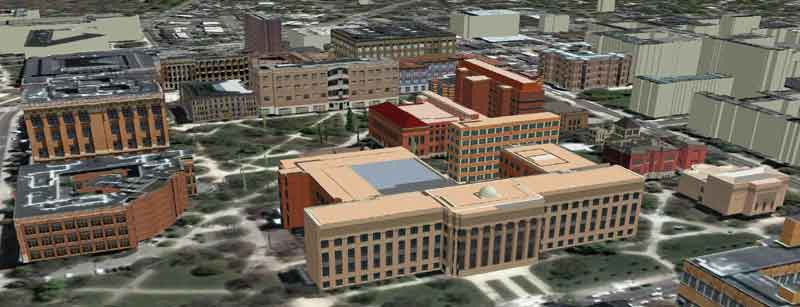Best viewed in Firefox browser (but should work in any current browser).  Works best with a high speed internet connection.
Final version of IMaGe logo created by Allen K. Philbrick from original artwork from the Founder.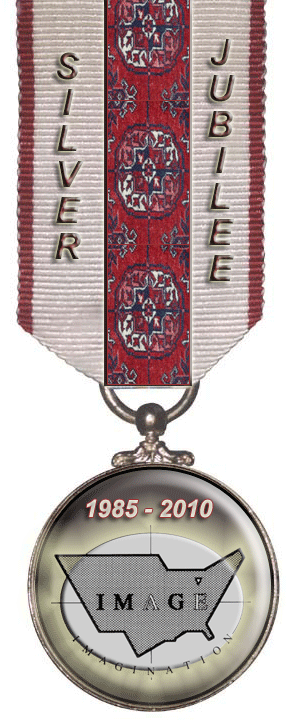Zipf's Hyperboloid--Revisited:
Sandra L. Arlinghaus, Ph.D., The University of Michigan
with input from an earlier article as part of an ongoing collaboration with
Michael Batty, Ph.D., University College London

Introduction

A set of cities may be rank-ordered according to population (or any number of other variables).  When it is, and the largest city is assigned the rank of 1, the next largest the rank of 2, and so forth, it follows that there is an inverse relationship between rank and population size.  Zipf characterized this relationship as the "rank-size rule."  The pattern inferred from plotting actual data corresponds to the equation xy=K which represents an hyperbola in the plane.  West, Brown, Enquist, and Savage characterize this rule as:

The empirically observed regularity is that settlements of rank r in the descending (size) array of settlements have a size equal to 1/r of the size of the largest settlement in the system. In other words, when the population size of towns is plotted against their frequency on a logarithmic scale, we see an approximately straight line with an exponent of -1. This relationship is known as the 'rank-size rule'.
By using log paper, they (as do most others) convert hyperbolae to straight lines.  A different, and more direct, approach involves viewing Zipf curves as hyperbolae and seeing them as part of a basic coordinate system on an hyperboloid of two sheets (see Solstice (June 2006) Zipf's Hyperboloid).

THE HYPERBOLOID OF TWO SHEETS

Part of the rationale for plotting on log paper and converting to straight lines is that lines are easier than curves to handle.  Such conversion, however, implies a functional relationship that may or may not be there.  Why convert curves as models, whose equations are known, to what they in fact are not?  Log paper masks the true shape of the curves.  Instead, Arlinghaus wished to look for a surface from which to derive these curves, as a set, in order to understand, rather than to mask, fundamental geometric structure.

The branches of the hyperbola in the 1st and 3rd quadrants may be rotated about the line y=x to generate a surface of revolution composed of two dish or bowl-shaped objects facing outward from the origin; follow this link and scroll down to find an image containing an hyperboloid of two sheets that the user can manipulate ("sheets" is the 3-dimensional equivalent of the 2-dimensional "branches").

To ease visualization of the pattern of intersecting planes with the hyperboloid sheets, consider the shapes in an upright position.  In analogy with the sphere, one sees, looking at the animations in Figure 1, that the parallels are circular sections sliced by the cutting plane in Figure 1a and that the meridians are hyperbola of varying curvature (Figure 1b) reminiscent of the pattern in the Zipf plot in its hyperbolic representation.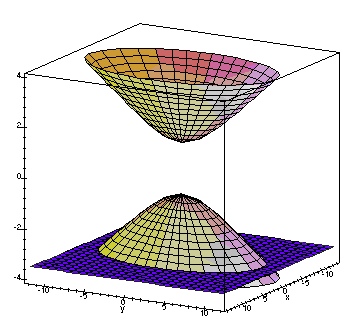Figure 1a.  Reproduced with permission of Putz, John F.  Animated Demonstrations for Multivariable Calculus.  Found at: http://archives.math.utk.edu/ICTCM/EP-10/C9/html/paper.html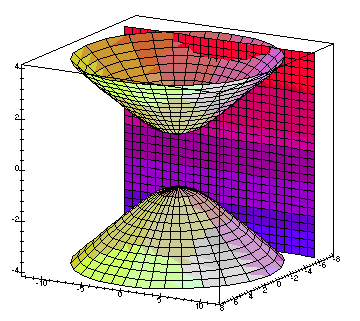Figure 1b.  Reproduced with permission of Putz, John F.  Animated Demonstrations for Multivariable Calculus.  Found at: http://archives.math.utk.edu/ICTCM/EP-10/C9/html/paper.html

The center of the solid, in each case, is the origin of the three dimensional coordinate system.  Each solid has two poles:  on the sphere, these are opposite ends of a diameter of the surface.  On the hyperboloid, these are opposite ends of the axis of bilateral symmetry of the surface, piercing each bowl at its base.  The "great hyperbolae" on the hyperboloid pass through a pole; they serve as geodesics on the surface.  The "small hyperbolae" do not pass through a pole.

In terms of Zipf plots, the farther a small hyperbola is from a great hyperbola, as suggested in the animation in Figure 1b, the larger is the biggest city and all subsequent cities by rank in the smaller hyperbola.  When the planes are parallel, visualization is easy in either two or three dimensions.  When they are not, and curves cross each other in the plane, visualization on the three dimensional surface permits visual disentanglement of form.

MAPPING THE HYPERBOLOID OF TWO SHEETS

When the hyperboloid of two sheets is orthographically mapped to the plane (through the origin) the visualization is as follows.  First, imagine that the plane is extended to include points at infinity so that the concept of parallel lines is removed.  The Euclidean space is converted to a non-Euclidean one.  Now, orthographic projection from the origin sends the upper sheet to the interior of a disk whose boundary is composed of the points at infinity:  what had been unbounded become compressed inside a single disk.   Points on the boundary of the disk are places where the hyperbolae touch the asymptotes (at infinity).  In this model, a great hyperbola, a geodesic passing through a pole (bottom of the bowl), maps to a diameter of the disk; small hyperbolae map to circular arcs with arc endpoints on the disk boundary (images in this link suggest how some of these mappings occur).  This systematic mapping of the hyperboloid of two sheets carries the viewer into the non-Euclidean world of hyperbolic geometry. The disk is the "Poincaré" disk.  This hyperbolic geometry model served as the base for Lobachevsky's geometry which has often been realized in relation to space-time problems in a variety of disciplines.

ZIPF'S HYPERBOLOID?

If Lobachevsky's hyperboloidal geometry is a natural base from which to drop Zipf curves, as Zipf's Hyperboloid, then how might that geometry be useful in understanding the emergence, timing, and decay of these systems?  Figure 2 shows mappings of Zipf curves in a compact form in the Poincaré disk.  Because the hyperbolae that pass a pole on the hyperboloid of revolution of two sheets map to diameters of the Poincaré Disk model of the hyperbolic plane, one has the freedom in the Zipf model to choose any Zipf hyperbola as the one about which to perform the revolution.  Thus, one might tie such choice to cultural or other considerations.  Suppose one wished, for example, to consider pre- and post-World War II patterns.  Then, 1950 might be selected as a date about which to create the surface of revolution.  Thus, in Figure 2a, imagine that the red diameter represents a U.S.A. rank-size database from 1950, extrapolated according to some procedure to infinity in either direction.  Then, the red circles, each with 10 percent fill, on either side, represent the corresponding extrapolated U.S.A. rank-size curves on either side of 1950; those farther from the diameter are farther from 1950.  Further, in Figure 2b, imagine that the green diameter represents a European rank-size (extrapolated) database from 1950.  The green circles on either side of the diameter, each with 10 percent fill, represent the corresponding European rank-size (extrapolated) databases on either side of 1950.  These figures have both been drawn with no crossings of rank-size curves.  Were there crossings, the corresponding circles would have perimeters that intersect, affecting fill concentration (which might be used to suggest urban or other concentration).  They have also been drawn assuming data on both sides of the date selected for revolution; naturally, if no data were available on one side, then no fill would appear on that side.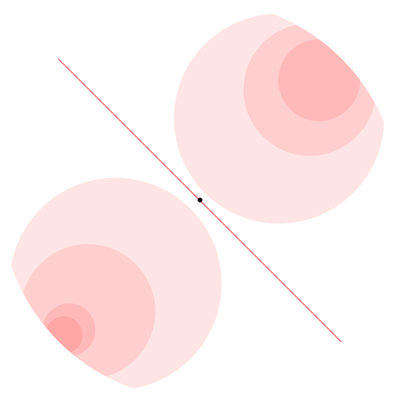Figure 2a.  Poincaré Disk with one set of rank-size curves.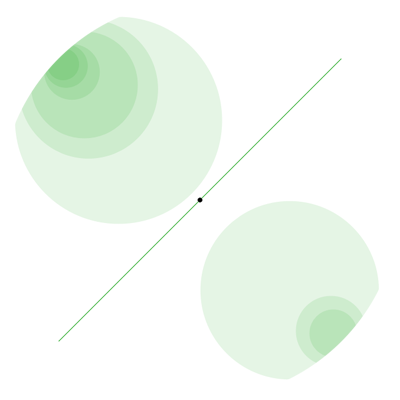Figure 2b.  Poincaré Disk with another set of rank-size curves.

When the two Disk layers are superimposed, as in Figure 3, one advantage to the compact Poincaré Disk model becomes clear:  Sets of rank-size curves from different parts of the world can be clearly visualized, simultaneously.  It is perhaps an interesting question to consider what overlapping regions might represent:  perhaps an opportunity for a Thiessen-style of transformation leading to rank-size art?  Indeed, the simple pattern in Figure 3 suggests the style of deeper pattern that can arise in association with complex issues:  it is the method of pattern creation so beautifully depicted in the art of Escher's Circle Limit series (inside Poincaré Disks).

Naturally, extrapolated rank-size curves need not be used; if instead, actual data only is used, the circles do not extend to the edge of the limit circle (points at infinity) of the Poincaré Disk.  We show the extrapolated curves here to indicate the power of the method.  Not only may it be used for the simultaneous display of complex datasets from disparate locations but also it handles finite as well as infinite processes.

 a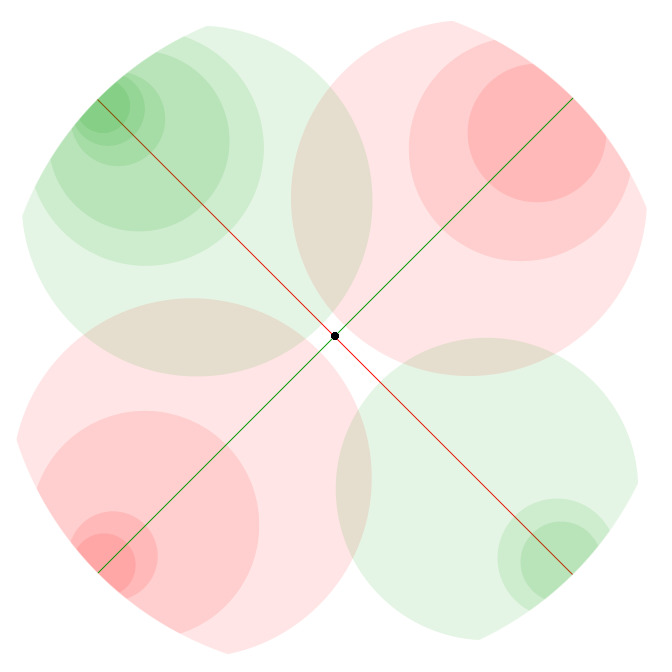Figure 3.  Poincaré Disk model of the hyperbolic plane with two sets of rank-size curves, from disparate locations, embedded.

Compression and Navigation:  the Appolonian Gasket and Fractal as Canonical Form in the Hyperbolic Plane

The layered image in Figure 3 is formed very simply from only a few Zipf-like curves.  While it is true that all Zipf curves might be represented in the hyperbolic plane wholly contained within the bounded, compact Poincaré disk, the pattern might become obscured by the complexity of introducing large sets of these curves.  If, however, one thinks of the sets of curves in Figure 4 as iterative pattern filling, or not filling, space, then it becomes possible to navigate the compressed forms.

Figure 4 shows a screen capture of a final frame in a movie (created by David Wright, see Indra's Pearls reference below).  Play the movie to see how sets of nested circles generate a fractal, called the Appolonian Gasket, shown as a yellow line in the movie.  One might suppose that different sequences of nested pattern (thought of as different rank-size curves in the hyperbolic plane, Poincaré disk model) would give rise to different Gaskets.  Indeed, from a Euclidean viewpoint, that is the case.  Here the advantage of using non-Euclidean geometry is of vital importance.  In the hyperbolic plane, all Appolonian Gaskets are in some sense "equivalent" (
Wikipedia.  Appolonian Gasket.  http://en.wikipedia.org/wiki/Apollonian_gasket).  Hence, the Appolonian Gasket is a canonical form for limiting position of sequences of Zipf curves in the hyperbolic plane (Poincaré Disk model)!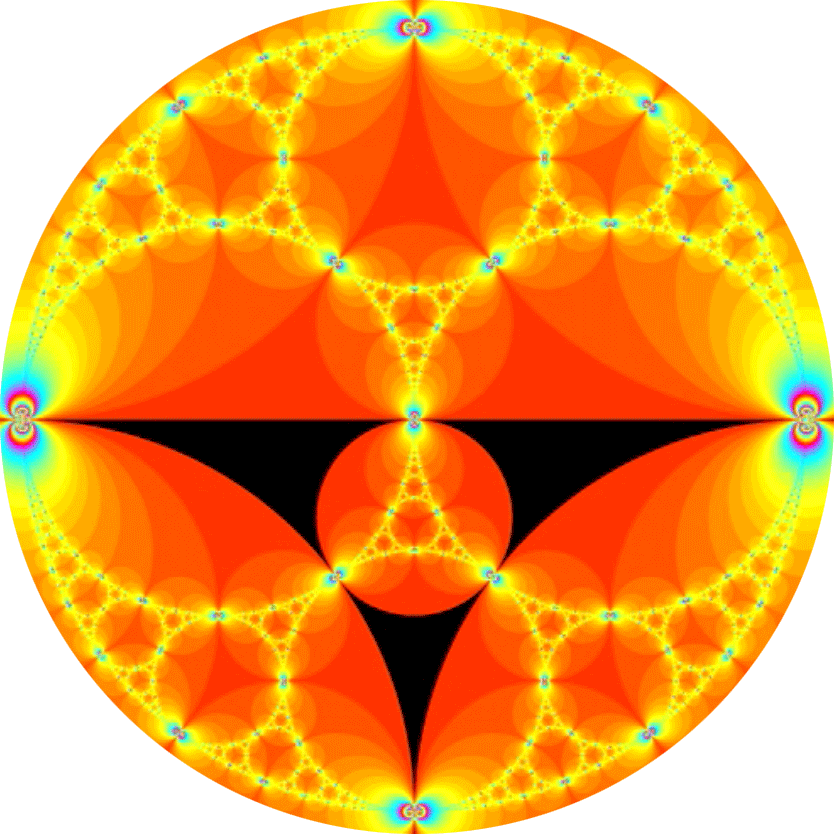Figure 4.  Screen capture from an image created by David Wright (see Indra's Pearls reference below).

What remains is to develop a systematic procedure for converting large data sets into sets of nested curves in the hyperbolic plane and then characterizing them as a Gasket.  Both of those processes are substantial endeavors.  However, the reason for using the hyperbolic plane is clear and the broad picture is set; what remains is to fill in systematic process.

Related Materials

• Arlinghaus, Sandra L. 1985.  "Fractals take a central place," Geografiska Annaler, 67B, pp. 83-88. Journal of the Stockholm School of Economics.
• Arlinghaus, Sandra L. and Arlinghaus, William C.  1989.  "The fractal theory of central place hierarchies: a Diophantine analysis of fractal generators for arbitrary Loschian numbers," Geographical Analysis: an International Journal of Theoretical Geography. Ohio State University Press. Vol. 21, No. 2, April, 1989; pp. 103-121.
• Arlinghaus, Sandra L. and Batty, Michael.  June 2006.  "Zipf's Hyperboloid?"  Solstice:  An Electronic Journal of Geography and Mathematics.  Ann Arbor:  Institute of Mathematical Geography.
• Batty, Michael.  29 May, 2003.  CUPUM '03:  Sendai, Japan.  The Emergence of Cities:  Complexity and Urban Dynamics.  Powerpoint display.  Contact the author: m.batty@ucl.ac.uk
• Coxeter, H. S. M.  1961, 1965 (fourth printing).  Introduction to Geometry.  New York:  John Wiley and Sons.
• Coxeter, H. S. M.  1961 (fourth edition). Non-Euclidean Geometry.  Toronto:  University of Toronto Press.
• Escher, M. C.  Circle Limit Series; see Peterson reference below.
• Harrison, Richard E.  Scientific American, cover, November, 1975, "Novel Map Projections."
• Putz, John F.  Animated Demonstrations for Multivariable Calculus.  Found at: http://archives.math.utk.edu/ICTCM/EP-10/C9/html/paper.html
• Peterson, Ivars.  2001.  Visions of Infinity.  Science News Online  Found at:  http://www.sciencenews.org/articles/20001223/bob8.asp
• Raisz, Erwin J.  1943.  Orthoapsidal world maps.  Geographical Review 33(1):  132-134.
• Snyder, John P.  1993.  Flattening the Earth:  Two Thousand Years of Map Projections.  Chicago:  University of Chicago Press.
• Thompson, D'Arcy W.  1917 (original publication date with others later).  On Growth and Form.  Cambridge University Press.
• Tobler, Waldo R.  1962.  A classification of map projections.  Annals of the Association of American Geographers 52(2):  167-175.
• West, Geoffrey; Brown, Jim; Enquist, Brian; and Savage, Van.  Network Dynamics:  Scaling and Distribution Networks.  Santa Fe Institute.  Found at:  http://www.santafe.edu/research/scalingNetworks.php
• Zipf, G.K. 1949. Human Behavior and The Principles of Least Effort, Addison Wesley, Cambridge, MA.

Author affiliations
Dr. Sandra Arlinghaus is Adjunct Professor at The University of Michigan, Director of IMaGe, and Executive Member, Community Systems Foundation.
Dr. Michael Batty is Bartlett Professor of Planning at University College London where he directs the Centre of Advanced Spatial Analysis.

 .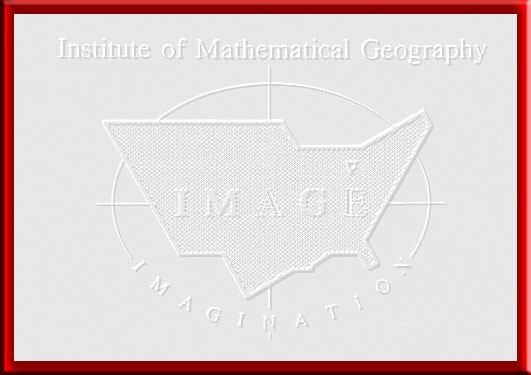Solstice:  An Electronic Journal of Geography and Mathematics,  Volume XXI, Number 1 Institute of Mathematical Geography (IMaGe). All rights reserved worldwide, by IMaGe and by the authors. Please contact an appropriate party concerning citation of this article: sarhaus@umich.edu http://www.imagenet.org http://deepblue.lib.umich.edu/handle/2027.42/58219 Solstice was a Pirelli INTERNETional Award Semi-Finalist, 2001 (top 80 out of over 1000 entries worldwide) One article in Solstice was a Pirelli INTERNETional Award Semi-Finalist, 2003 (Spatial Synthesis Sampler). Solstice is listed in the Directory of Open Access Journals maintained by the University of Lund where it is maintained as a "searchable" journal. Solstice is listed on the journals section of the website of the American Mathematical Society, http://www.ams.org/ Solstice is listed in Geoscience e-Journals IMaGe is listed on the website of the Numerical Cartography Lab of The Ohio State University:  http://ncl.sbs.ohio-state.edu/4_homes.html Congratulations to all Solstice contributors. Remembering those who are gone now but who contributed in various ways to Solstice or to IMaGe projects, directly or indirectly, during the first 25 years of IMaGe: Allen K. Philbrick | Donald F. Lach | Frank Harary | H. S. M. Coxeter | Saunders Mac Lane | Chauncy D. Harris | Norton S. Ginsburg | Sylvia L. Thrupp | Arthur L. Loeb | George Kish |
1964 Boulder Drive,
Ann Arbor, MI 48104
734.975.0246

http://deepblue.lib.umich.edu/handle/2027.42/58219
sarhaus@umich.edu